## Make Scroll Odds Accurate

### Make Scroll Odds Accurate

So, as I've been looking into the way the game runs and such, I've come to where I think I know how scrolls work for certain.

In game, scrolls are stated as increasing the odds of getting the scrolled role by 10 times (see: https://i.gyazo.com/f2426e0715adc19d617 ... 7ede42.png ). Also, interestingly, the odds listed on the role wheel go from 7% (as 1/15) to 42% (as 10/24), which isn't a 10x increase.
I've described in past posts that these 7% odds aren't even correct, but if they're going to remain incorrect, my primary suggestion is to fix the numbers so they're consistent. Either add more lots so that the odds go from 7% to 70%, or change the labels on scrolls to 6x odds.

As I'll describe in this post, however, none of the above would be fully correct. First, the odds of each role varies based on the role list in use. For example, in all-any, the odds of a particular player getting mafioso (without scrolls) is about 5%, while the odds of the same player getting vampire hunter (also without scrolls) is about 1.2%. Most are near 3%, the 1/33 expectation (as there are 33 roles). Second, when scrolls are applied, even only accounting for averages (i.e. not other players' scrolls), the odds of getting a particular role doesn't increase by 10 times. As with unscrolled, it varies by the role list in use, but taking all-any as an example, the odds of getting a role you're scrolled for only goes up about 4 times on average.

Clearly stated example: In all-any, without a scroll for arso, I'm told I have a 7% chance of getting it. However I only really have a 3% chance of getting it. I'm told if I scroll, my odds will increase by 10x to 42%. However my odds will only really increase by 4x to 12%.

The Suggestion: Fix the numbers displayed so they're correct, or else fix the way the roles are spun and handed out.

Now onto the math. For the sake of simplifying calculations and explanations, the role-list is all-any. Also, since this will calculate the average change as a result of scrolling, other players' scrolls will be safely neglected.

The probability of getting a role R is equal to the probability of getting R given that it was 1 of the 15 pre-spun, times the probability that it was pre-spun: P(R) = P(R|prespun)*P(prespun)

We can clearly see that P(R|prespun) is in linear proportion to P(R), so if P(R|prespun) doubles, so does P(R). Since our point of analysis (the change in probability) follows this linear proportion, we can disregard fully calculating P(R) and only focus on P(R|prespun).

Next, we can understand that the probability of getting R given that it's prespun is equal to the sum of the expected outcomes of each position which gets randomly given to spin for a role. To clarify, each player is given a position in a queue, and then spins the role wheel sequentially, picking from 1 of the 15 pre-spun roles. If I am the 1st person in this queue, every role on the pre-spun list is available to me. If I am the 2nd person, 14 of these roles are available to me, but I do not know which role was removed.

Simplified: P(R|prespun) = P(picking 1st)*E(lotRatio | picking 1st) + P(picking 2nd)*E(lotRatio | picking 2nd) + ... + P(picking 15th)*E(lotRatio | picking 15th)

E(lotRatio | picking nth) represents the expected ratio of my lots for R to the total lots for R, given that I'm in the nth position in queue.
For example, if I'm the 1st person in queue, and nobody has any scrolls, the expected lotRatio is 1/15.

We will simplify this as a function LR(n).

LR(n) is not too hard to find a pattern for.
When not scrolling:
LR_ns(1) = 1/15
LR_ns(2) = (1/15)(0) + (14/15)(1/14) = 1/15
LR_ns(3) = (1/15)(0) + (14/15)( (1/14)(0) + (13/14)(1/15) ) = (14/15)(13/14)(1/13) = 1/15
When scrolling:
LR_s(1) = 10/24
LR_s(2) = (1/15)(0) + (14/15)(10/23)
LR_s(3) = (1/15)(0) + (14/15)( (1/14)(0) + (13/14)(10/22) ) = (14/15)(13/14)(10/22)

LR_s(n) generalizes as such: https://i.gyazo.com/145e3dd29bb5646d75b ... bd0834.png
LR_ns(n) always equals 1/15.

And now, inserting LR for each case, we finally get two equations to put in proportion.
When not scrolling: P_ns(R|prespun) = (1/15)( LR_ns(1) + LR_ns(2) + ... + LR_ns(15) ) = (1/15)( (15)(1/15) ) = 1/15
When scrolling: P_s(R|prespun) = (1/15)( LR_s(1) + LR_s(2) + ... + LR_s(15) )

Thus the linear effect of a scroll is: https://i.gyazo.com/219ed1a7f44ab65a7e2 ... c8df46.png

This means that in all-any, on average, equipping a scroll will only increase the probability of receiving that role by 4.04818 times, not 10.After-Unity™

luls:
Spoiler: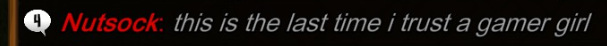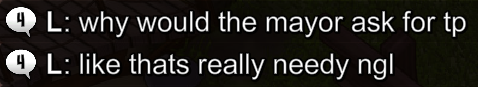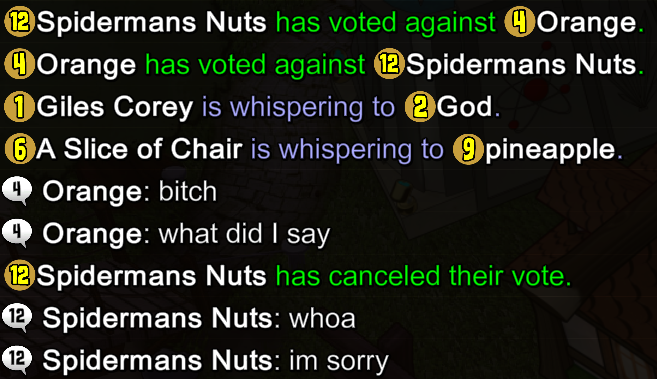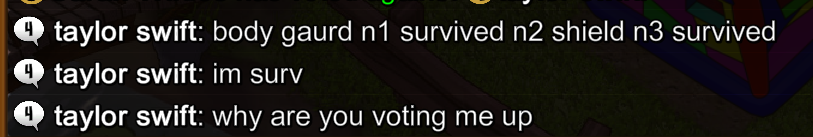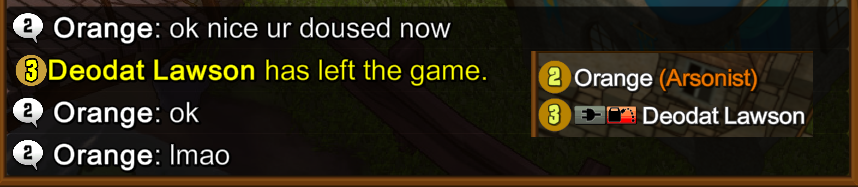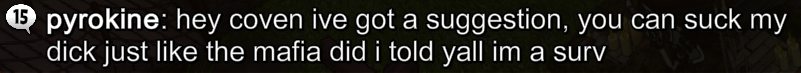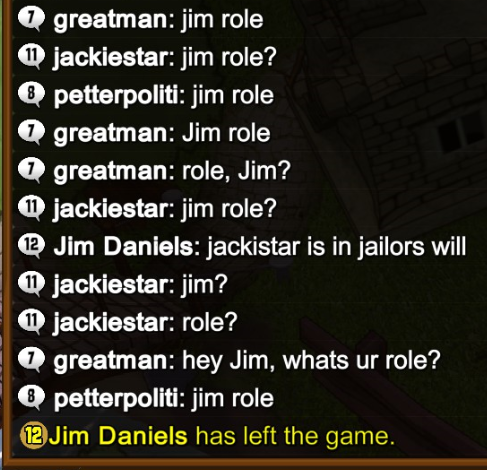Superalex11
InvestigatorPosts: 267
Joined: Fri Jan 08, 2016 10:11 pm

### Re: Make Scroll Odds Accurate

My scrolls seem to work fine in role list matches, maybe thats what the %s are based on? I mean, theres only 1 mode without a role list. When they dont work,for a few games in a row, theres usually 3 or 4 other players scrolling for the same role in the same match
goosegoosegoosegoosegoose
Spoiler:Spoiler:kyuss420
GodfatherPosts: 1537
Joined: Sat Aug 19, 2017 1:33 am
Location: Im here